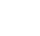Project URA-HPC

Many problems of paramount importance in diverse fields can be described by matrices and their direct solution involves the computation of some function over these matrices. Examples are: solution of integer or fractional partial differential equations; analysis of complex networks; etc.

For many real instances , classic algorithms cannot be employed as they do not scale well to solve these problems. Specifically, intrinsic data-dependencies make them not amenable for large-scale parallelization. Hence, in practice people resort to alternative methods to arrive at some solution, in most cases an approximate solution. O\$en, it is even hard to gauge the quality of this approximation.

We aim to make fundamental contributions to a method for computing matrix functions based on their Neumann series, consisting of weighted sums of powers of the matrix . Moreover, we use a Monte Carlo algorithm where a matrix to the power k is computed using random walks of length k over the matrix . Monte Carlo methods have the preeminent facet of being embarrassingly parallel, achieving a high degree of efficiency on a parallel system. In our method, each walk is independent from one another and can be computed completely in parallel. This class of algorithms positions itself as the most appropriate to exploit the computational power of new exascale machines to the fullest. A second, crucially important feature of this Monte Carlo method, that sets it apart from the classic algorithms, is that it allows calculating individual entries of the result, avoiding the computation of the entire matrix. While real- world matrices are typically sparse, the result matrix will, in general, be dense. Thus, for large problems the representation of the output matrix in memory is in itself unfeasible, rendering its computation using classic methods impractical. With our approach we can, for instance, compute the trajectory of a single state variable in a dynamic system (eg, the voltage of a node in an electrical network) or the communicability of a single node in a network.

However, there are two major limitations of Monte Carlo methods. First, the handling of very large instances requires the splitting of the matrix across machines which implies that the walks may need to be continued on another machine. This may entail a significant communication overhead that needs to be aggressively mitigated. The second is that these methods suffer from a very slow convergence rate, hence possibly implying a large number of walks for the desired result precision.

In this research project we propose to address these two problems, thus bringing Monte Carlo methods to the forefront as enablers of solutions for up to now intractable problems. We have some initial work on the first of these problems , where a distributed implementation of the method was developed that is able to hide communication costs by aggregating walks in asynchronous messages. Still, a more effective solution should be possible, namely by taking advantage of more recent tool features [5,35], such as tasking with data-dependencies and support for tasks in accelerators.

We also have recent work that addresses a specific instance of the second problem. We have recently made important contributions [1,2] on adapting multilevel techniques to improve the convergence rate of the Monte Carlo when computing the exponential of a matrix. In this proposal, we aim to study whether this approach can be further optimized and extended to other matrix functions, and we plan to explore other variance reduction techniques to improve the overall convergence rate of the method.

In yet another research avenue, we have found that using this Monte Carlo approach with some modifications we are able to efficiently solve systems with derivatives of fractional order. Fractional calculus has a wide range of practical applications, eg , and has not yet seen a more widely spread adoption due to its intrinsic computational difficulty. Our expectation is that our idea can have a broad impact and be a significant contribution from this project, and we plan to apply it to both Magnetic Resonance Imaging (MRI) and the modeling of chemical reactions .

We propose to demonstrate the usefulness of our method by using it to solve two other highly relevant and current world problems. One is the analysis of complex networks, namely to compute the communicability of a network and the centrality of a node. The first is related to the exponential of the adjacency matrix of the network and the latter to the inverse of that matrix.

The other area in which an effective method of determining the exponential of a matrix can be a game changer is in the analysis of dynamic systems. Solving the matrix exponential of the system may allow the direct computation of the system state at a given time, and hence that of any system output at any time in the future, instead of having to simulate the system over time as is done today.